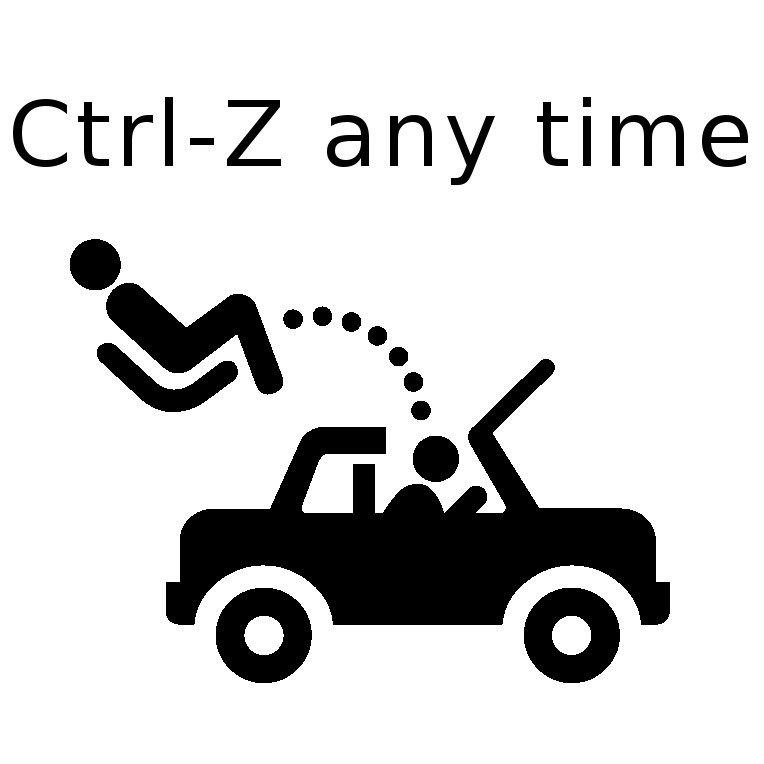# Edexcel A level maths 9MA0 2018 paper 3: interactive! (with mouse)

### Hover the mouse over a vector for guidance.ARecommended Resource

Main page - loads of interactive A level mechanics

6.We can integrate the given velocity function to 'recover' the displacement function that it 'came from' (through differentiation, as velocity is the instant-by-instant gradient of displacement).

It can be convenient to calculate the definite integral between limits 1 and 4; but it can also be instructive to form a general displacement function in terms of t, by using the known displacement at t = 1 to determine the constant of integration in each component, as shown in the algebra.

Now we can represent the displacement function as a vector parallelogram, and follow the path of the particle as t varies (going from (-2, 2) at t = 0, through the origin (A) at t = 1, and through (2, -30) at t = 4. (Hold 'shift' while left click-and-dragging the tip of the resultant vector.)

Apply Pythagoras to find the magnitude of the vector at t = 4.

Your browser does not support the HTML5 canvas tag.

load a saved work file or a background image file:

7.

(a) The only forces acting horizontally are the friction, which is at its maximum value of 0.14 times the magnitude of the reaction of the ground against the box, and the horizontal component of the tension, which a triangle or (even better!) a parallelogram will show to be four fifths of the 40, because of the 3-4-5 right angled triangle. The reaction force is equal to the weight (20g) of the box minus the vertical lift from the tension, which is three fifths (the triangle again) of 40.

Apply Newton's second law. ma, the force with which the crate is moving - its rate of increase in momentum - will be equal to the sum total of the forces applying to it, i.e. what's left of the 32N component of the tension after deducting the magnitude of the friction which is acting the other way.

So the equation is: 20a = 32 - 0.14(20g - 24), which you can solve for a.

(b) Now the vertical reaction at the ground is against the sum (instead of the difference) of the weight and the vertical component of the push, and you can explain the effect this will have on the friction and, in turn, on the acceleration.

Your browser does not support the HTML5 canvas tag.

load a saved work file or a background image file:

8.

(a) As in no.6, we need to integrate velocity to recover the displacement function, but this time constant acceleration means that the suvat (s = ut + ½at2) has done that job. So we can use the given velocity at the origin (for u) and the given position at t = 2 (for s) to solve for a, as shown.

(b) Now we use another suvat (v = u + at) to find each component of the velocity at the moment when the particle is at A, and then again for a general velocity function for the motion subsequently. See how the bottom of the straight red line on the graph shows the velocity when the particle is at A. Hold shift and (left) drag the tip of the resultant to consider how the velocity and each of its components vary with time.

When the two components are equal (pointing NE) you can solve for t.

Your browser does not support the HTML5 canvas tag.

load a saved work file or a background image file:

9.

(a) Drag the top-left vector parallelogram (hover the mouse near but not quite where the three vectors join, then left-click and drag) and land it so the corner is at B... thus sense how the tension in the string is like that of vertical and horizontal strings pulling with forces whose magnitudes are in proportion to the drawn lengths. In particular, the vertical component of the tension (three fifths of it) is what you need to calculate a moment about A. Because it's perpendicular to the point B's displacement from A.

Now move the group of rotatable moments so their join is at A. (And rotate! Left click and drag the grey circle at the middle of a moment.) See how equilibrium means that the two clockwise moments must equal the anticlockwise one created by the vertical component of tension acting at B. Solve for T (the tension).

(b) Put the horizontal component of tension (four fifths of it) equal to 2MG (because they are the only forces involved in the horizontal equilibrium).

(c) Move the moments away from A. (Or just use control-Z to revert to the initial state.) Move the other vector parallelogram to A, and consider all the forces acting vertically, in order to calculate the force with which the wall is lifting the beam at A. Then calculate vertical over horizontal, which will be the tan of the angle of the resultant of the two (from the horizontal).

Your browser does not support the HTML5 canvas tag.

load a saved work file or a background image file:

Main page - more interactive A level mechanics

ARecommended Resource
Garden City Maths - Online tuition!Today! Just open a meeting in Zoom and send me an invite by email (below). Or email me.
Balloon Calculus ... Don't integrate - balloontegrate!

email: tom@ballooncalculus.org.